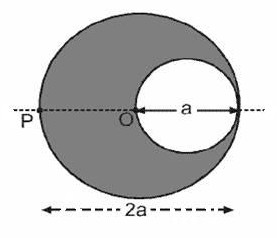Friday, May 25, 2012

IIT JEE 2012 Questions on Magnetic Field due to Infinitely Long Current Carrying Cylinders

“The world is a dangerous place, not because of those who do evil, but because of those who look on and do nothing.”
– Albert Einstein.

Today we will discuss two questions pertaining to the magnetic field produced by infinitely long current carrying cylinders. The first question is the usual single correct answer type multiple choice question. The second one is an integer answer type question, the answer to which is a single digit integer ranging from 0 to 9. Here are the questions with their solution.
(1) An infinitely long conducting cylinder with inner radius R/2 and outer radius R carries a uniform current density along its length. The magnitude of the magnetic field B‌ as a function of the radial distance r from the axis is best represented by‌‌Case (i): < R/2
Using Ampere’s circuital law we find that the magnetic fiel at points at distance r < R/2 is zero.
[We have ∫B.d = μ0I.
Since the current passing through the surface enclosed by the path of integration is zero, the value of B is zero at distance r < R/2]
‌‌Case (ii)
For distance r lying between R/2 and R (or, R/2 ≤ r < R) we have
∫B.d = μ0r2J π(R/2)2J] where J is the current density
r2J π(R/2)2J is the current passing through the surface enclosed by the path of integration in this case]
Or, B×2πr = μ0r2J π(R/2)2J]
This gives B = 0J/2)[r – (R2/4r)]
Case (iii)
For r R we have
B.d = μ0R2J π(R/2)2J]
Or, B×2πr = μ0R2J π(R/2)2J]
This gives B = 0J/2r)[R2 – (R2/4r)] = 3μ0JR2/8r
The graph shown in option (D) indicates the above three cases correctly.(2) A cylindrical cavity of diameter ‘a’ exists inside a cylinder of diameter 2a as shown in the figure. Both the cylinder and the cavity are infinitely long. A uniform current density J flows along the length. If the magnitude of the magnetic field at the point P is given by N μ0 aJ/12, then the value of N is
If the cylinder has no cavity, the magnetic flux density B1 at the point P is given by
∫B1.d = μ0πa2J
Therefore, B1×2πa = μ0πa2J
Or, B1 = μ0aJ/2
Since there exists a cavity, the current is reduced by π(a/2)2J and the magnetic field is reduced by B2.
The field B2 is given by
∫B2.d = μ0π(a/2)2J
Therefore, B2×2π(3a/2) = μ0π(a/2)2J
[The circular path of integration in this case has radius a+(a/2) = 3a/2]
Or, B2 = μ0aJ/12
The magnitude B of the magnetic field at the point P due to the actual conductor with the cavity is given by
B = B1 B2 =  μ0aJ/2μ0aJ/12
Or, B = 5μ0aJ/12
In the question the above field is given as Nμ0aJ/12
Therefore N = 5.

Wednesday, May 09, 2012

AIPMT Main Exam – Two Questions Involving Discharge of a Capacitor Through an Inductor

"Live as if you were to die tomorrow. Learn as if you were to live forever."
– Mahatma Gandhi

Questions appearing in AIPMT Main (Physics) question paper are generally not as simple as those appearing in AIPMT Preliminary question paper. Those who get qualified (by passing the preliminary examination) should bear this in mind and prepare accordingly. I give below two questions involving the discharge of a charged capacitor through an inductor:
(1) A condenser of capacity C is charged to a potential difference of V1. The plates of the condenser are then connected to an ideal inductor of inductance L. The current through the inductor when the potential difference across the condenser reduces to V2 is
(1) [C(V1 V2)2/L]1/2
(2) C(V12 V22)/L
(3) C(V12 + V22)/L
(4) [C(V12 V22)/L]1/2
The above question appeared in AIPMT Main 2010 question paper.
The initial energy of the charged capacitor is ½ CV12. When the potential difference across the capacitor reduces to V2  the energy is ½ CV22. Therefore, the energy lost by the capacitor is ½ C(V12 V22). This amount of energy is gained by the inductor.
If I represents the discharge current at the instant when the potential difference across the capacitor reduces to V2 we have
½ C(V12 V22) = ½ LI2
[Remember that the energy of an inductor carrying a current I is ½ LI2 and the energy is store in the magnetic field]
From the above equation we obtain
I = [C(V12 V22)/L]1/2
(2) Capacitance of 6 μF is charged by 6 V battery. Now it is connected with inductor of 5 mH. Find the current in the inductor when 1/3rd of total energy is magnetic.
This question was asked as half the part of a free response (and not a multiple choice) question in the AIPMT Main 2007 question paper.
[Current practice is to ask objective type (multiple choice) questions].
The initial energy E1 of the charged capacitor is given by
E1 = ½ CV2 = ½ ×(6×10–6)×62
Since one-third of total energy is mentioned as magnetic in the question, we understand that the energy of the inductor carrying the discharge current I is E1/3.
Therefore we have
E1/3 = ½ LI2
Or, (1/3) ×[½ ×(6×10–6)×62] = ½ ×(5×10–3)I2
This gives I = 0.12 A